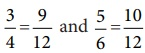Home | | Maths 8th Std | Comparison of rational numbers

# Comparison of rational numbers

When two integers or fractions are given, we know how to compare them and say which is greater or smaller. Now, in the same way, we can compare a pair of rational numbers.

Comparison of rational numbers

It is useful to remember the following points:

• Every positive number is greater than zero.

• Every negative number is smaller than zero.

• Every positive number is greater than every negative number.

• Every number on the right of a number on a number line is greater than that number.

When two integers or fractions are given, we know how to compare them and say which is greater or smaller. Now, in the same way, we can compare a pair of rational numbers.

Type 1 : Comparing two rational numbers with opposite signs

Example 1.3

Compare 5/17 and -10/19.

Solution:

Since every positive number is greater than every negative number, we conclude that 5/17 > -10/19.

Type 2 : Comparing two rational numbers represented by two fractions with same denominators

Example 1.4

Compare 1/3 and 4/3.

Solution:

Since the denominators are the same, just compare the numerators.

Since 1 < 4, we conclude that 1/3 < 4/3.

Type 3 : Comparing two rational numbers represented by two fractions with different denominators

Example 1.5

Compare 3/4 and 5/6.

Solution:

The LCM of the denominators is 12 (Find it!). Consider for each rational number an equivalent rational number with the LCM 12 as denominator

We get3/4 = 9/12 and 5/6 = 10/12, which become like fractions now.

Here, 9/12 < 10/12. Hence, we conclude that 3/4 < 5/6.

Type 4 : Comparing two rational numbers that are not in standard form

Example 1.6

Compare 9/-4 and -2/3.

Solution:

The number 9/-4 is not in standard form. First put it in the standard form.Now, we shall compare the fractions -9/4 and -2/3. We find that these two fractions are unlike fractions. To make them as like fractions, we make use of their LCM, which is 12.

We can now compare their equivalent fractions -9/4 = -27/12 and -2/3 = -8/12 (How?)

We find that the denominators are the same and so just comparing the numerators –27 and –8 are enough Visualizing these numbers on the number line, we see that–8 is to the right of –27 and hence (–8) > (–27). This leads to the result that -8/12 > -27/12 and consequently we conclude that -2/3 > 9/-4 .

Example 1.7

Write the following rational numbers in ascending and descending order.Solution:

First make the denominators to be positive and write the numbers in standard form as. Here, the LCM of 5,10,15,20 and 30 is 60 (Find it!). Change the given rational numbers in equivalent form with common denominator 60.Comparing the numerators alone, that is, 36, 42, 45, 28 and –32 we see that

45 < −42 < −36 < −32 < −28Also, its reverse order gives the descending order asTags : Numbers | Chapter 1 | 8th Maths , 8th Maths : Chapter 1 : Numbers
Study Material, Lecturing Notes, Assignment, Reference, Wiki description explanation, brief detail
8th Maths : Chapter 1 : Numbers : Comparison of rational numbers | Numbers | Chapter 1 | 8th Maths